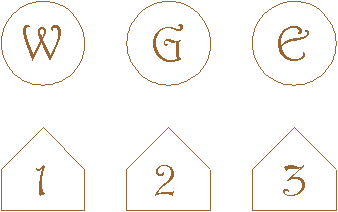Connect the three houses to the three utilities without crossing paths.
Lately, I've had quite a few people ask me about the Water, Gas, Electricity problem.  The graph involved is known as K(3,3) -- The complete graph connecting three points (W G E) to three points (1 2 3).  A graph is called planar when it can be drawn without crossing paths.  This graph, K(3,3), is non-planar.  It cannot be drawn without crossing some paths.  I felt obliged to provide proof.

Proof One.  A beauty sent by John Conway.  The following cycle exists in the graph: (1 W 2 G 3 E).Three more connections are needed: 1 G, 2 E, and 3 W.  By the Pigeonhole Principle, at least two connections must be drawn inside or outside.  These two connections must cross.  Therefore, K(3,3) is nonplanar.

Proof Two.  Euler proved that connected planar graphs satisfy the following important relation:

vertices + faces - edges = 2
With 6 vertices and 9 edges, there must be 5 faces.  This means that the plane would be divided into 5 regions.  Consider each of the following 9 cycles: (1 E 3 G), (E 3 G 2), (3 G 2 W), (G 2 W 1), (2 W 1 E), (W 1 E 3), (2 W 3 E), (2 G 1 E), (1 W 3 G).  Each of these cycles is closed, so each one of these would make a region, or face.  Since a planar K(3,3) would divide the plane into 9 regions, it does not satisfy Euler's equation, and thus is not planar.

Proof Three, sent to me by Richard Guy.  Again, use Euler's formula, then note that  K(3,3)  is bipartite, so that any region in a drawing without crossings has an even number of edges, hence at least 4.   The five faces have at least 20 edges.  Each edge may be shared by two faces, so at least 10 edges are in the graph.  But the graph has 9 edges  --  contradiction.

With tricks, the Water-Gas-Electricity problem can be solved.  Just run a line under one of the houses.

Joseph DeVincentis had the following comments:

"There's a problem on your page this week with Proof Two for why
the W-G-E problem has no solution.

The 9 cycles you describe in this proof are not necessarily
"faces" per the definition used in Euler's formula.  Some
of them could exist but have other points both inside and
outside of them.

For instance, take Conway's diagram from proof 1 and connect
3 to W inside the loop and 2 to E outside.  This diagram has
five of these "faces" even though it only has eight edges.
Four of these (1W3E, 2W3G, 2G3E, and 2W1E) are actual faces
(the last of them being the "outside" face), but 2W3E cannot
be considered a face by any means."